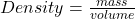## A teacher performing a demonstration finds that a piece of cork displaces 23.5 cm3 of water. The piece of cork has a mass of 5.7 g. What is

Question

A teacher performing a demonstration finds that a piece of cork displaces 23.5 cm3 of water. The piece of cork has a mass of 5.7 g. What is the density of the cork

in progress 0
6 months 2021-07-29T20:41:05+00:00 1 Answers 42 views 0

Density = 0.243 g/cm³

Explanation:

Volume of cork = 23.5 cm³

Mass = 5.7 g

To find the density of the cork;

Density can be defined as mass all over the volume of an object.

Simply stated, density is mass per unit volume of an object.

Mathematically, density is given by the formula;Substituting into the formula, we have;

Density = 5.7/23.5

Density = 0.243 g/cm³